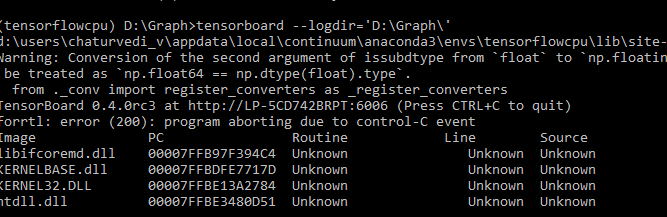# keras-Tensorboard

Many times we need to visualize our model on Tensorboard, for this we have to save our model and at runtime check out the performance. Here is the code for a simple linear regression using Keras and tensorboard.

import Libraries:

```import keras
import numpy as np
from keras.models import Sequential
from keras.layers import Dense
from keras.wrappers.scikit_learn import KerasRegressor
from keras.callbacks import TensorBoard```

Let’s take two lists of numbers,

```X=np.array([1,2,3,4,5,6,7,8,9,10,12,11,22,21,21,12,12,8,7,5,4,6,7])
Y=np.array([1,2,3,4,5,6,8,8,9,10,13,11,22,21,21,10,12,8,7,5,4,5,7])```

Create a neural network Model,

```from keras.wrappers.scikit_learn import KerasRegressor

def baseline_model():
model = Sequential()
# Compile model
tensorboard = TensorBoard(log_dir="D:\Graph".format(time()))
return model
```

Build a regressor model,

```from time import time
tensorboard = TensorBoard(log_dir="D:\Graph".format(time()))
estimator = KerasRegressor(build_fn=baseline_model, epochs=110, batch_size=1,verbose=1, callbacks=[tensorboard])
```

predict a value,

`estimator.predict(np.array())`
```1/1 [==============================] - 0s
```
`array(11.940549850463867, dtype=float32)`

Score will be,

```score = estimator.score(X, Y)
```

Now, how can we visualize the model ?. Go to tensorboard….The visualization will be like this,

Nice Articles for tensorflow: